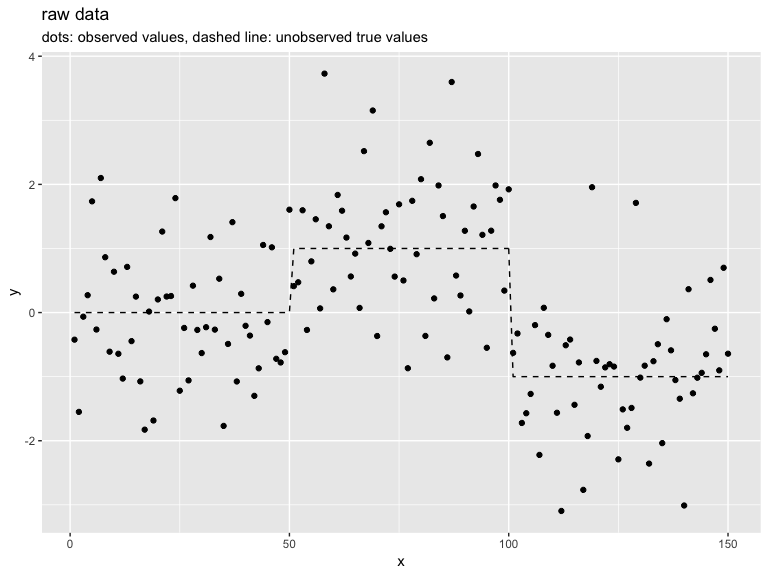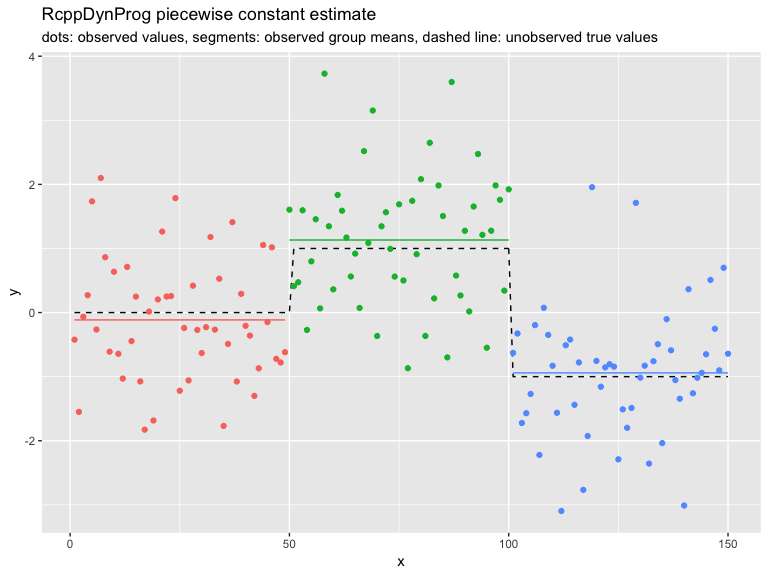# Segmentation

#### 2019-02-02

In this example we fit a piecewise constant function to example data.
Please see here for a discussion of the methodology.

library("RcppDynProg")

plot <- requireNamespace("ggplot2", quietly = TRUE)
if(plot) {
library("ggplot2")
}

set.seed(2018)
g <- 50
d <- data.frame(
x = 1:(3*g)) # ordered in x
d$y_ideal <- c(rep(0, g), rep(1, g), rep(-1, g)) d$y_observed <- d$y_ideal + rnorm(length(d$y_ideal))

if(plot) {
plt1 <- ggplot(data= d, aes(x = x)) +
geom_line(aes(y = y_ideal), linetype=2) +
geom_point(aes(y = y_observed)) +
ylab("y") +
ggtitle("raw data",
subtitle = "dots: observed values, dashed line: unobserved true values")
print(plt1)
}As a heuristic, we set our regularization penalty to a value that treats permuted data (no relation between x and y) as a single partition.

y_permuted <- d$y_ideal[sample.int(nrow(d), nrow(d), replace = FALSE)] solve_with_penalty <- function(ycol, penalty) { n <- length(ycol) indices = seq_len(n) x <- const_costs(ycol, 1+numeric(n), 1, indices) x <- x + penalty solve_interval_partition(x, n) } lb <- 1 ub <- 10 while(length(solve_with_penalty(y_permuted, ub))>2) { ub <- ub*2 } while(TRUE) { mid <- ceiling((ub+lb)/2) if(mid>=ub) { break } si <- solve_with_penalty(y_permuted, mid) if(length(si)<=2) { ub <- mid } else { lb <- mid } } print(ub) ##  5 We now use this penalty to segment the data. Notice we recover the actual problem structure. soln <- solve_with_penalty(d$y_observed, ub)
print(soln)
##    1  50 101 151
d$group <- as.character(findInterval(d$x, soln))
group_means <- tapply(d$y_observed, d$group, mean)
d$group_mean <- group_means[d$group]

print(sum((d$y_observed - d$y_ideal)^2))
##  151.876
print(sum((d$group_mean - d$y_ideal)^2))
##  2.973557
if(plot) {
plt2 <- ggplot(data= d, aes(x = x)) +
geom_line(aes(y = y_ideal), linetype=2) +
geom_point(aes(y = y_observed, color = group)) +
geom_line(aes(y = group_mean, color = group)) +
ylab("y") +
ggtitle("RcppDynProg piecewise constant estimate",
subtitle = "dots: observed values, segments: observed group means, dashed line: unobserved true values") +
theme(legend.position = "none")
print(plt2)
}The same solution through the more succinct solve_for_partitionc() interface.

# x_cuts <- solve_for_partition(d$x, d$y_observed)
# sometimes a different penalty due to problem chunking
x_cuts <- solve_for_partitionc(d$x, d$y_observed, penalty = ub)
print(x_cuts)
##     x       pred group  what
## 1   1 -0.1147501     1  left
## 2  49 -0.1147501     1 right
## 3  50  1.1321951     2  left
## 4 100  1.1321951     2 right
## 5 101 -0.9412289     3  left
## 6 150 -0.9412289     3 right
d$estimate <- approx(x_cuts$x, x_cuts$pred, xout = d$x, method = "constant", rule = 2)$y d$group <- as.character(findInterval(d$x, x_cuts[x_cuts$what=="left", "x"]))

print(sum((d$y_observed - d$y_ideal)^2))
##  151.876
print(sum((d$estimate - d$y_ideal)^2))
##  2.973557
print(sum((d$estimate - d$y_observed)^2))
##  147.9564
if(plot) {
plt3 <- ggplot(data= d, aes(x = x)) +
geom_line(aes(y = y_ideal), linetype=2) +
geom_point(aes(y = y_observed, color = group)) +
geom_line(aes(y = estimate, color = group)) +
ylab("y") +
ggtitle("RcppDynProg piecewise constant estimate",
subtitle = "dots: observed values, segments: observed group means, dashed line: unobserved true values") +
theme(legend.position = "none")
print(plt3)
}